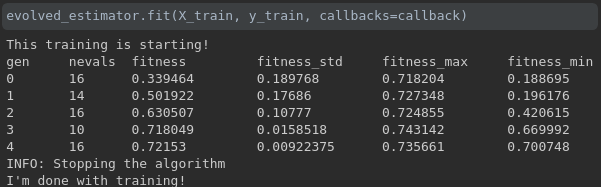# Custom Callbacks

sklearn-genetic-opt comes with some pre-defined callbacks, but you can make one of your own by defining a callable with certain methods.

## Parameters

The callback must be a class with inheritance from the class `BaseCallback` that implements the following methods:

on_start: This is evaluated before starting the generation 0, it should return `None` or `False`. It expects the parameter estimator.

on_step: This is called at the end of each generation, the result of them must be a bool, `True` means that the optimization must stop, `False`, means it can continue. It expects the parameters record, logbook and estimator.

on_end: This method is called at the end of the las generation or after a stopping callback meets its criteria. It expects the parameters logbook and estimator, it should return `None` or `False`.

All of those methods are optional, but at least one should be defined.

## Example

In this example, we are going to define a dummy callback that stops the process if there have been more than N fitness values bellow a threshold value.

The callback must have three parameters: record, logbook and estimator. Those are a dictionary, a deap’s Logbook object and the current `GASearchCV` (or `GAFeatureSelectionCV`) respectively with the current iteration metrics, all the past iterations metrics and all the properties saved in the estimator.

So to check inside the logbook, we could define a function like this:

```N=4
metric='fitness'
threshold=0.8

def on_step(record, logbook, threshold, estimator=None):
# Not enough data points
if len(logbook) <= N:
return False
# Get the last N metrics
stats = logbook.select(metric)[(-N - 1):]

n_met_condition = [x for x in stats if x < threshold]

if len(n_met_condition) > N:
return True

return False
```

As sklearn-genetic-opt expects all this logic in a single object, we must define a class that will have all these parameters, so we can rewrite it like this:

```from sklearn_genetic.callbacks.base import BaseCallback

class DummyThreshold(BaseCallback):
def __init__(self, threshold, N, metric='fitness'):
self.threshold = threshold
self.N = N
self.metric = metric

def on_step(self, record, logbook, estimator=None):
# Not enough data points
if len(logbook) <= self.N:
return False
# Get the last N metrics
stats = logbook.select(self.metric)[(-self.N - 1):]

n_met_condition = [x for x in stats if x < self.threshold]

if len(n_met_condition) > self.N:
return True

return False
```

Now, let’s expend it to add the others method, just to print a message:

```from sklearn_genetic.callbacks.base import BaseCallback

class DummyThreshold(BaseCallback):
def __init__(self, threshold, N, metric='fitness'):
self.threshold = threshold
self.N = N
self.metric = metric

def on_start(self, estimator=None):
print("This training is starting!")

def on_step(self, record, logbook, estimator=None):
# Not enough data points
if len(logbook) <= self.N:
return False
# Get the last N metrics
stats = logbook.select(self.metric)[(-self.N - 1):]

n_met_condition = [x for x in stats if x < self.threshold]

if len(n_met_condition) > self.N:
return True

return False

def on_end(self, logbook=None, estimator=None):
print("I'm done with training!")
```

So that is it, now you can initialize the DummyThreshold and pass it to in the `fit` method of a `GASearchCV` instance:

```callback = DummyThreshold(threshold=0.85, N=4, metric='fitness')
evolved_estimator.fit(X, y, callbacks=callback)
```

Here there is an output example of this callback:Notice that there is an extra INFO message, that is general for all the callbacks that stops the training.